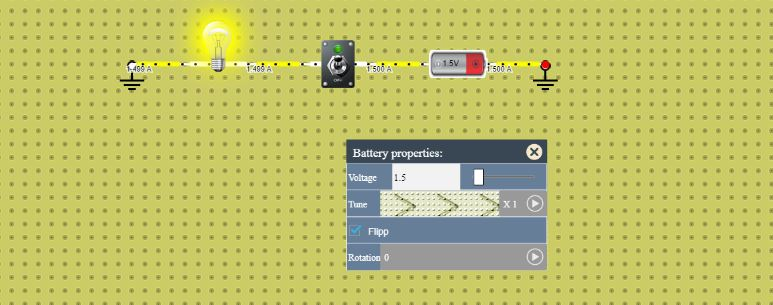# How to use Battery in Lab

You have to follow steps in order to use the battery in DCACLab.

## Steps to use Battery in Lab

Step 1: Navigate through the list of the devices/components and locate the symbol of battery as shown in image below.Step 2: Click on the icon of the battery as shown in the image above or grab the symbol, a battery will appear on the below board.Step 3: Connect the battery in your circuit as per your need.### Controlling the properties of the battery

While working on the circuit click on the battery, a Gear like icon will appear near to the battery as shown in the image below.Click on the gear icon and the whole property of the battery will be shown which you can control and change as per your circuit need.You can control the voltage of the battery and you can flip as well as rotate it as per your requirement.

#### Components in a Battery Property1. Here you can change the voltage of the battery, you can either enter put the desired voltage in the box or you can swipe the scroll bar in order to set the voltage of the battery upto the desired volts.
2. In this part you are able to control the fraction value of voltage. The section has options to vary the voltage of battery in mili volt, micro volt and nano volt.
3. By using the check box you can flip the battery at its position in order to turn the terminals to opposite.
4. In this you can rotate the battery, you can rotate it from 0° to 90°, 180° and 270°.

You can practice working with the battery on the circuit simulator.

### What is a Battery

In general a Battery is defined as the combination of two or more cells. A cell is a device which stores electrical power, the energy stored in the form of chemical energy which is then converted into electrical energy with the help of chemical reactions in order to create the flow of electron.

The Battery of a cell has three main components.

• Annode (Negative side of the battery)
• Cathode (Positive side of the battery)
• Electrolyte (A substance which reacts with the annode and cathode in order to create current flow)

### Connecting batteries in parallelAbove image shows the resultant voltage across the load when we connect the two batteries in parallel. As per the circuit connection shown above if the two battery of 1.5V and 2.5V are connected in parallel then the resultant voltage will become 2V.

Note : E = (E1 R2 + E2 R1) / (R1 + R2)

Where,

E = Resultant voltage

E1 = Voltage of the first battery

R1 = Internal resistance or the first battery

E2 = Voltage of the second battery

R2 = Internal resistance or the second battery

### Connecting batteries in seriesAbove image shows the resultant voltage across the load when we connect the two batteries in series. As per the circuit connection shown above if the two battery of 1.5V and 2.5V are connected in series then the resultant voltage will become the sum of both batteries voltage that is 4V.

Note : E = E1 + E2

where,

E = Resultant voltage

E1 = Voltage of the first battery

E2 = Voltage of the second battery

### Battery polaritiesAbove screenshot demonstrates that how the polarity effects the direction of the rotation of fan in the Lab.

### Comparison of AC and DC signalsIn the above figure shows the difference between the AC voltage source and the battery using the oscilloscope in the lab.

### Changing the voltage of the batteryAbove image shows that how the change in voltage of the battery reflects in the circuit. By using multimeter the voltage of the battery is measured.

•
•
•
•
•
•